Levels »

### The Pit, Elevation: -15.3m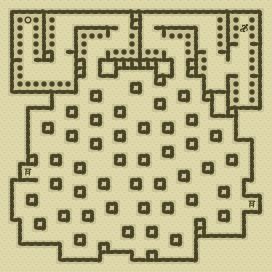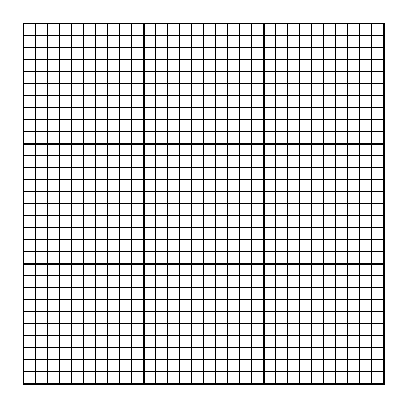### Items

•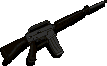Broken M-16 (29, 2) (on Greg)
•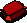2 Sapphires (16, 12) - (17, 17)
•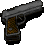Colt .45 Pistol (29, 2) (on Greg)
•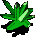Green Crystal (16, 30) (30 uses left)

•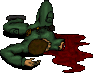(29, 2) Greg
•Broken M-16
•Colt .45 Pistol

### Monsters

•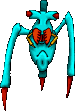45 Skitters (Random rate: 4) (19, 1) - (14, 2) - (14, 3) - (29, 3) - (29, 3) - (14, 4) - (20, 4) - (21, 4) - (20, 5) - (26, 6) - (26, 7) - (2, 8) - (8, 11) - (23, 11) - (22, 12) - (3, 13) - (8, 14) - (6, 15) - (18, 16) - (15, 17) - (25, 17) - (29, 17) - (18, 18) - (12, 20) - (15, 20) - (29, 20) - (4, 23) - (10, 23) - (11, 23) - (15, 24) - (18, 24) - (3, 25) - (14, 26) - (16, 26) - (19, 26) - (11, 27) - (3, 28) - (13, 28) - (16, 28) - (16, 28) - (14, 29) - (15, 29) - (16, 29) - (18, 29) - (19, 30)
•2 Sentinels (10, 7) - (21, 7)
•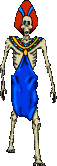9 Ghasts (Random rate: 2) (29, 1) - (2, 2) - (14, 2) - (17, 8) - (29, 10) - (24, 12) - (19, 28) - (13, 29) - (16, 30)

### Locations

• 6 Ladder-guarding lizard triggers (4, 17) - (7, 20) - (28, 21) - (4, 22) - (25, 24) - (27, 27)
• 2 Bomb-guarding lizard triggers (20, 10) - (23, 12)
• 3 Crystal-guarding lizard triggers (16, 26) - (13, 28) - (19, 29)
• 5 Crossing-guard lizard triggers (14, 11) - (15, 14) - (15, 17) - (17, 20) - (21, 23)
• Exploding pod hiding nuclear device (29, 11)
• 2 South-facing Sentinel barriers (10, 7) - (21, 7)
• 33 Exploding pods (24, 3) - (7, 4) - (28, 5) - (5, 8) - (11, 9) - (17, 9) - (22, 9) - (25, 9) - (7, 11) - (4, 13) - (19, 13) - (6, 14) - (26, 14) - (10, 15) - (12, 16) - (21, 16) - (28, 17) - (4, 19) - (23, 20) - (10, 22) - (17, 23) - (28, 23) - (1, 24) - (9, 24) - (27, 24) - (11, 25) - (23, 25) - (2, 28) - (22, 28) - (7, 29) - (17, 29) - (8, 30) - (20, 30)
• Save rune(2, 1)